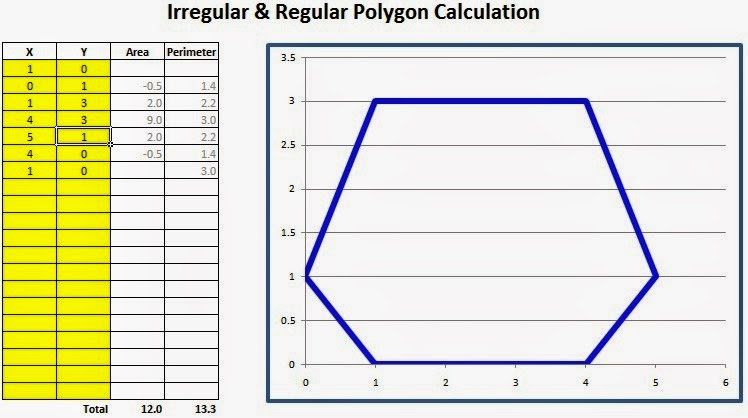## Wednesday, December 18, 2013

### Liquid Volume In Complicated Horizontal Cross Section Tank

Some audiences asked about how to calculate liquid volume in a horizontal tank which have a complex cross section like a tank on tanker truck, tank which has cross section shaped like a rectangle but with smaller top smaller, curved cross section of all sides, and rounded all corners, like the one on photo.

If the horizontal cross section of the tank is uniform (homogeneous) for the whole length of tank, then the volume of liquid in the tank is calculated by: liquid covered cross-sectional area multiplied by length of tank.

The question is how to calculate the cross-sectional area which is covered by liquid ??

In my opinion, the easiest way is to photograph the tank cross section, and put the photo onto graph paper. The more boxes on that graph paper the more accurate calculation results, see figure below. You can make graph paper using Excel sheet, and put tank cross section photo on to it, like I did.

When taking the picture, the camera position must be perpendicular to the cross section of tank and camera aims right around the midpoint of tank cross section. It is better to photograph the tank from a distance, and use telephoto or zoom function on the camera to make tank looks bigger.

If the photo is taken from a short distance, and the tank for example in a higher position of the camera, then the camera will seeing up. It will make the bottom of the tank appear larger than actual, and the top of tank will look smaller than it really is.

Liquid level in the tank can be measured using a dipstick (measuring stick). The measurement results are described on to a graph paper in order to obtain x and y coordinates. More coordinates (x, y), or more boxes on the graph paper, means more accurate calculation result.

For curved line of polygon, more coordinates means more fine the arch so that more accurate calculation result. For a straight line like the one representing liquid surface, then only 2 point coordinates are needed, because mathematically a straight line is a line connecting two points.

To calculate the cross sectional area of ​​the tank, can be done with an irregular polygon area calculation. Please read polygon area article.

Download Excel program here, to calculate the area of ​​polygon of tank cross section. After you get coordinates from graph paper, then input those coordinates in to the Excel sheet containing the program. Below is a simple example of Excel program that automatically calculates polygon area with certain coordinates.See the above example of a simple calculation, polygon cross-sectional area of ​​the tank can be read in the column 'Area' and in the row 'Total', the number is 12.0.

A straight line at top of polygon is representing liquid surface inside the tank. The surface height was measured by dipstick, as the above example: liquid surface height is 3 units of measurement.

Based on scale system, if each number representing the x and y coordinate with distance of 20 cm, the calculated area by the program must be multiplied by 400 or = 20x20, in order to obtain area units of measurement square centimeters (cm2). If each x and y number represents 1 cm, then calculation result will be square centimeters (cm2), no need to multiplication.

Data row on the Excel program can be added by 'Copy - Insert Copied Cells' function, so the more the coordinates can be calculated and calculation result will be more accurate.

As we can obtain the cross-sectional area of ​​the tank that is covered by liquid, then cross-sectional area multiplied by the length of the tank will become the volume of liquid in the tank.

Accuracy of calculation will also depend to the thickness of tank plate, tank level position, and others.